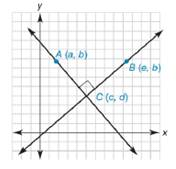Chapter 10.2, Problem 42EElementary Geometry For College St...

7th Edition
Alexander + 2 others
ISBN: 9781337614085

Solutions

Chapter
SectionElementary Geometry For College St...

7th Edition
Alexander + 2 others
ISBN: 9781337614085
Textbook Problem

Find an equation involving a , b , c , d , and e if A C ⃡ ⊥ B C ⃡ .(HINT: Use slopes.)To determine

To find:

An equation involving a, b, c, d, and e if ACBC.Explanation

By theorem,

If two lines are perpendicular, then the product of their slopes is -1 or one slope is negative reciprocal of the other slope.

(i.e) If l1l2, then m1.m2=-1 or m2=-1m1

Here ACBC, thus we have to find the slopes for the two lines AC and BC.

Then by using the theorem, we can make the required equation.

The slope of the line that contains the points x1,y1 and x2,y2 is given by

m=y2-y1x2-x1 for x2x1

Let mAC- and mBC- are the slopes of the line AC- and BC- respectively.

The given vertices are Aa, b, and Cc, d.

Using the slope formula and choosing x1=a, x2=c, y1=b, and y2=d.

mAC-=d-bc-a

The given vertices are Be, b and Cc, d

Still sussing out bartleby?

Check out a sample textbook solution.

See a sample solution

The Solution to Your Study Problems

Bartleby provides explanations to thousands of textbook problems written by our experts, many with advanced degrees!

Get Started